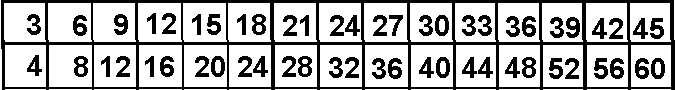Multiple Strips & Fraction Bars

Yah, I know it looks like times tables printed the wrong way, but, it provides multiples of numbers, and that's what you need. Click on the graphic at the left then use your browser to print two copies.

Multiple strips and fraction bars are a means of making the ideas of multiple, equivalent fractions, and the addition or subtraction of fractions more concrete.

DIGITAL MANIPULATIVES

Experiment digitally with these manipulatives.  See strips.xls.

Make Strips

After printing the two copies of the graphic above, you need to cut out strips. Cut out strips the LONG WAY.

Each strip represents the multiples of the first number on the strip. The 1-strip has multiples of one up through fifteen. The 2-strip, begins with 2, has the multiples of two up through thirty.

Shown just below are the 3s strip and the 5s strip.The multiples of 3 (numbers 3 through 45) are on one stnp. Multiples of five, 5 through 75, are on the 5s strip.

Activity: Finding Common Multiples

To find the multiples of two or more whole numbers from 1 to 13 (since you have 13 different strips), select the appropriate strips and compare the numbers on each strip to find common multiples.

Using the 3-strip and the 5-strip, one may note that 15, 30, and 45 are common to both since only those numbers are found on both strips: Fifteen, thirty and forty-five (45 is the last number on the 3-strip) are multiples of both three and five. There exist other common multiple of 3 and 5, but they are too large for these strips.Using the 3-strip and the 4-strip, one may note that 12, 24, and 36 are the only numbers from one through forty-five (45 is the last number on the 3-strip) which are multiples of both three and four.Fraction Bars

Since each strip represents multiples of a number, a pair of strips used together parallel to each other may be used to represent multiples of fractions or equivalent fractions. To create fraction strips, choose a numerator strip and a denominator strip. Place the numerator strip “above” and parallel to the denominator strip.

Activity: Writing Equivalent Fractions

Choose a fraction so that the numerator and denominator are whole numbers from 1 to 13. Use the corresponding multiple strips to write equivalent forms of this fraction by placing the numerator-strip parallel to and “above” the denominator-strip so the ends match up. The three-fourths strips is shown.© 2009, Agnes Azzolino www.mathnstuff.com/math/spoken/here/2class/60/mult.htm